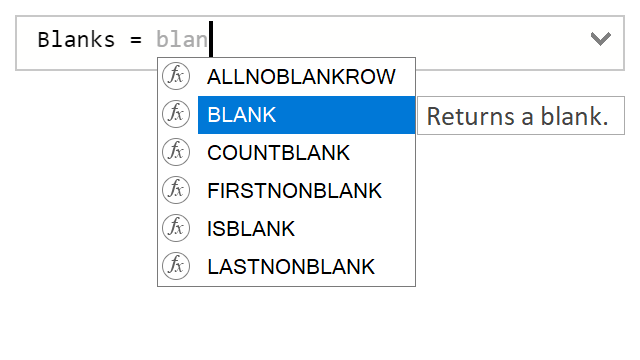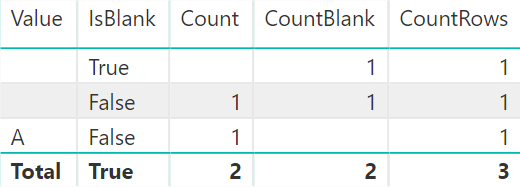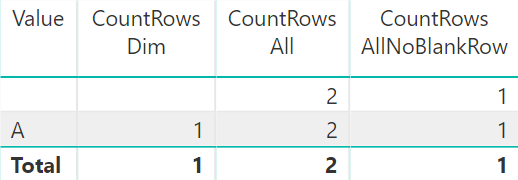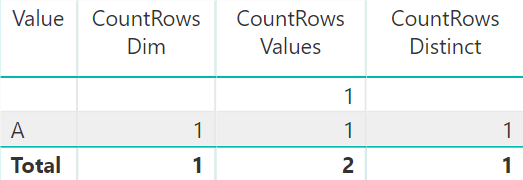# Different blanks in DAXThere are several DAX functions that deal with blanks. Surprisingly, some of them have a different idea of a blank. In this article, I am covering these discrepancies.

### BLANK

The BLANK function returns a blank value. If you don’t know what a blank is, I suggest you to read an excellent article by Marco Russo: Handling BLANK in DAX. In short, it is important to understand that a blank value in DAX behaves differently from null in M or NULL in SQL. Also, the following expressions all evaluate to TRUE:

``````BLANK () = BLANK ()  // TRUE
BLANK () = ""        // TRUE
BLANK () = 0         // TRUE
BLANK () = FALSE ()  // TRUE``````

### ISBLANK

The ISBLANK function checks if a value is blank. It is strict in what it considers a blank: only true blanks will result in the function returning TRUE:

``````ISBLANK ( BLANK () )  // TRUE
ISBLANK ( "" )        // FALSE
ISBLANK ( 0 )         // FALSE
ISBLANK ( FALSE () )  // FALSE``````

### FIRSTNONBLANK/LASTNONBLANK

Both FIRSTNONBLANK and LASTNONBLANK are also strict in their definition of a blank: they return the first or last non-blank value, respectively. They don’t consider empty strings, zeros, or FALSE to be blanks.

### COUNTBLANK

Here’s something that surprised me recently: the COUNTBLANK function counts not only true blanks, but also empty strings.

Consider the following calculated table:

``````Table =
{
BLANK (),
"",
"A"
}``````

And the following measures:

``````IsBlank    = ISBLANK ( SELECTEDVALUE ( 'Table'[Value] ) )
Count      = COUNT ( 'Table'[Value] )
CountBlank = COUNTBLANK ( 'Table'[Value] )
CountRows  = COUNTROWS ( 'Table' )``````

If we put everything in a table visual in Power BI, this is what we will see:Note how both COUNT and COUNTBLANK count empty strings, meaning their combined result is not guaranteed to be the same as COUNTROWS.

### ALLNOBLANKROW

This function, even though it has “BLANK” in its name, deals with the virtual blank row, not scalar blanks.

When two tables are in a one-to-many relationship and the table on the many side has values that do not exist in the table on the one side, a virtual blank row will be added to the table on the one side. This row can be seen under certain circumstances.

To illustrate this phenomenon, consider the same table as in the previous section together with the following one:

``Dim = { "A" }``

As well as the following measures:

``````CountRows Dim           = COUNTROWS ( Dim )
CountRows All           = COUNTROWS ( ALL ( Dim ) )
CountRows AllNoBlankRow = COUNTROWS ( ALLNOBLANKROW ( Dim ) )``````

If we relate the two tables and use Dim[Value] together with the new measures in a Power BI table visual, we will get the following result:As you can see, the ALLNOBLANKROW function does not return the virtual blank row, while ALL does. The reason why we see the value of 1 for the blank row is that ALLNOBLANKROW removes the filter on Dim.

In this regard, the behavior of ALLNOBLANKROW corresponds to DISTINCT, which also does not include the virtual blank row, though it respects the current filter context. The behavior of ALL corresponds to VALUES. You can see it in the following image:Note: be aware that under certain conditions, DISTINCT behaves completely differently from the other three functions — ALLNOBLANKROW, ALL, and VALUES. This topic is outside of scope of this blog post — maybe Marco or Alberto have covered it somewhere, or maybe I will when I grow up and be like them. If you are curious, read my comment about it on SQLBI: Avoiding circular dependency errors in DAX.

### DISTINCTCOUNTNOBLANK

In contrast to DISTINCTCOUNT, DISTINCTCOUNTNOBLANK excludes simple blanks from its count, although it includes the virtual blank row.

• Jes Hansen

Hi Daniil,
It may interest you to learn that the IN operator applies strict comparison as opposed to the relational operators =,>,,>= you use in the blog post.

EVALUATE
CALCULATETABLE ( VALUES ( ‘Table'[Value] ), ‘Table'[Value] IN { “” } )
will return only { “” }

whereas
EVALUATE
CALCULATETABLE ( VALUES ( ‘Table'[Value] ), ‘Table'[Value] = “” )
will return { “”, BLANK() }

and
EVALUATE
CALCULATETABLE ( VALUES ( ‘Table'[Value] ), NOT ‘Table'[Value] IN { “” } )
returns { BLANK(), “A” }

whereas
EVALUATE
CALCULATETABLE ( VALUES ( ‘Table'[Value] ), ‘Table'[Value] “” )
returns only {“A”}

The same principle applies to zero (0). So if you were to have a table Table = { Blank(), 0, 85 } you would see the same pattern if you swapped “” with 0 in the conditions of the above DAX queries.

I don’t believe Marco and Alberto have updated their article to reflect this. The IN operator was introduced after they originally wrote the article. Perhaps it will be covered in their new edition of The Definitive guide to DAX.

Best regards Jes.

• That’s a very good comment, Jes — thank you!

• Frank Tonsen

Unfortunately, there’s more: In Excel 2016 the DAX function COUNTBLANK does not count empty strings.
(BTW, the Excel function does count empty strings.)